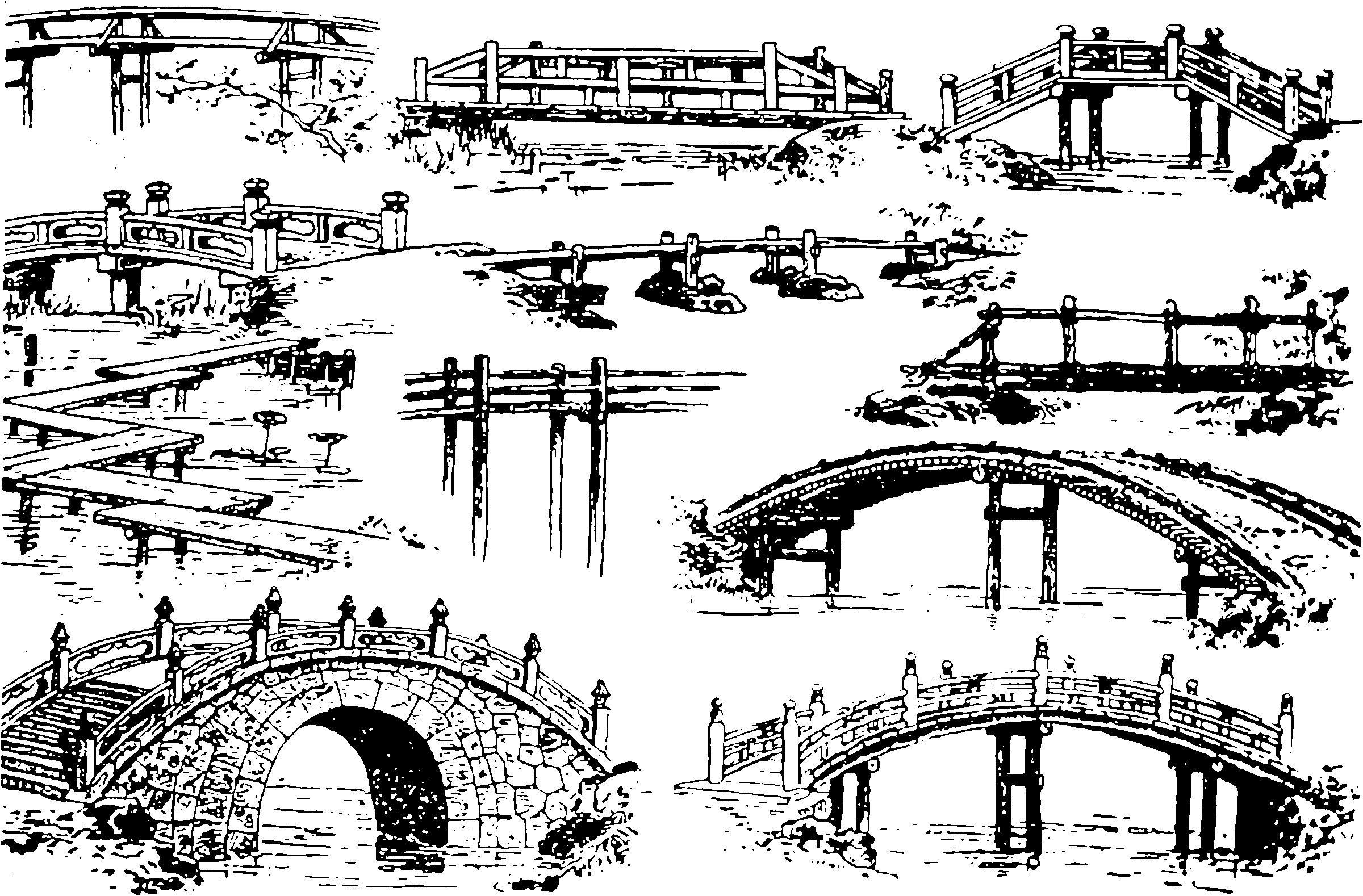# Markov bridge processes

## Especially Lévy bridges, Doob h-transforms

$$\renewcommand{\var}{\operatorname{Var}} \renewcommand{\dd}{\mathrm{d}} \renewcommand{\pd}{\partial} \renewcommand{\bb}{\mathbb{#1}} \renewcommand{\bf}{\mathbf{#1}} \renewcommand{\vv}{\boldsymbol{#1}} \renewcommand{\mm}{\mathrm{#1}} \renewcommand{\cc}{\mathcal{#1}} \renewcommand{\oo}{\operatorname{#1}} \renewcommand{\gvn}{\mid} \renewcommand{\II}{\mathbb{I}} \renewcommand{\disteq}{\stackrel{d}{=}} \renewcommand{\rv}{\mathsf{#1}} \renewcommand{\vrv}{\vv{\rv{#1}}} \renewcommand{\Ex}{\mathbb{E}} \renewcommand{\Pr}{\mathbb{P}}$$

A bridge process for some time-indexed Markov process $$\{\rv{x}(t)\}_{t\in[0,T]}$$ is obtained from that process by conditioning it to attain a fixed value at the final time $$\rv{x}(T)=Y$$ starting from $$\rv{x}(0)=X$$, on an interval $$[0,T]$$. We write that as $$\{\rv{x}(t)\mid \rv{x}(0)=X,\rv{x}(T)=Y\}_{t\in[0,T]}.$$ Put another way, given the starting and finishing values of a stochastic markov process, I would like to rewind time to find out the values of its path at a midpoint which is “compatible” with the endpoints.1 Or, if we jump back then forward again, we can construct generalised autoregressive processes.

## Lévy bridges

I am mostly interested in bridges for Lévy incremenet processes in particular.

It is trivial (more or less) to derive the properties of the bridge for a Wiener process, so that can be found in every stochastic processes textbook. There is an introduction for Lévy processes in . TODO: check that it admits processes with jumps.

Fitzsimmons, Pitman, and Yor (1993) describes a general assortment of methods for handling the properties of bridges, and Perman, Pitman, and Yor (1992) specialises them on pure jump processes (Poisson, gamma).

🏗In fact “bridge” is a terrible metaphor for these processes and it has simplified nothing for me to use this image as an illustration I am so sorry.

### When is a Lévy bridge distribution computationally tractable?

I know it is simple for Gamma, Brownian, and Poisson Lévy processes. Are there others? Yor (2007) asserts that among the Lévy processes, only Brownian and Gamma processes have closed form expressions for the bridge. That is not right; a Poisson process has a simple bridge (binomial sub-division of the increment). Also, both Gamma and Brownian can admit a deterministic drift term.

However, I cannot think of other easy examples. Compound Poisson processes, for example, will have a nasty integral term over an unbounded number of dimensions if we do it the obvious way.

Surely there are proofs about this? I have half a mind to see if I cannot find a classification of processes admitting tractable bridges with my rudimentary variational calculus skills.

A classic. TBC.

## General Markov processes

i.e. not just process with stationary increments, but satisfying the Markov property.

Alexandre Hoang Thiery describes Doob’s h-transform method for general Markov processes and notes that while it might be good for deducing certain properties of the paths of such a conditioned process, it is not necessarily a good way of sampling it. Although if you happen to derive an analytic form that way, it is good for both.

For a fairly old and simple concept, work in the area skews surprisingly recent (i.e post 1990), given that applications seem to cite the early, gruelling as the foundation; perhaps for about 30 years no-one wanted to learn enough potential theory to make it go? Or perhaps I am missing something because of e.g. terminology drift.